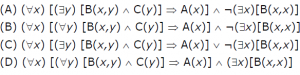# GATE | GATE-CS-2003 | Question 71

Consider the following logic program P
A(x) <- B(x, y), C(y)
<- B(x,x)
Which of the following first order sentences is equivalent to P?

``````

(A) A
(B) B
(C) C
(D) D